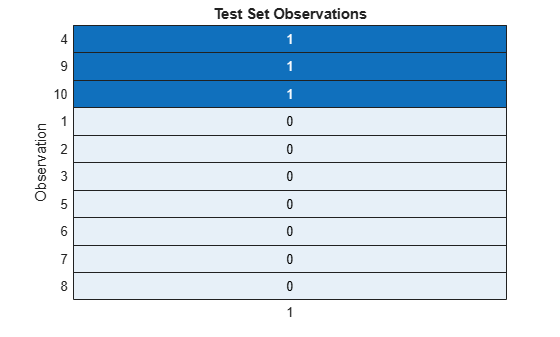# test

Test indices for cross-validation

## Syntax

``idx = test(c)``
``idx = test(c,i)``
``idx = test(c,"all")``

## Description

example

````idx = test(c)` returns the test indices `idx` for a `cvpartition` object `c` of type `'holdout'` or `'resubstitution'`. If `c.Type` is `'holdout'`, then `idx` specifies the observations in the test set.If `c.Type` is `'resubstitution'`, then `idx` specifies all observations. ```
````idx = test(c,i)` returns the test indices for the repetitions specified in `i` of a `cvpartition` object `c` of type `'kfold'` or `'leaveout'`. If `c.Type` is `'kfold'`, then `idx(:,j)` specifies the observations in test set `i(j)`.If `c.Type` is `'leaveout'`, then `idx(:,j)` specifies the observation reserved for testing at repetition `i(j)`. ```

example

````idx = test(c,"all")` returns the test indices for all repetitions of a `cvpartition` object `c`. Column j in `idx` indicates the observations in test set j for an object of type `'kfold'` or `'leaveout'`. (since R2023b)```

## Examples

collapse all

Identify the observations that are in the test (holdout) set of a `cvpartition` object.

Partition 10 observations for holdout validation. Select approximately 30% of the observations to be in the test set.

```rng('default') % For reproducibility c = cvpartition(10,'Holdout',0.30)```
```c = Hold-out cross validation partition NumObservations: 10 NumTestSets: 1 TrainSize: 7 TestSize: 3 IsCustom: 0 ```

Identify the test set observations. Observations that correspond to 1s are in the test set.

`holdout = test(c)`
```holdout = 10x1 logical array 0 0 0 1 0 0 0 0 1 1 ```

Visualize the results. The fourth, ninth, and tenth observations are in the test set.

```h = heatmap(double(holdout),'ColorbarVisible','off'); sorty(h,'1','descend') ylabel('Observation') title('Test Set Observations')```Identify the observations that are in the test sets, or folds, of a `cvpartition` object for 3-fold cross-validation.

Partition 10 observations for 3-fold cross-validation. Notice that `c` contains three repetitions of training and test data.

```rng("default") % For reproducibility c = cvpartition(10,"KFold",3)```
```c = K-fold cross validation partition NumObservations: 10 NumTestSets: 3 TrainSize: 7 6 7 TestSize: 3 4 3 IsCustom: 0 ```

Identify the test set observations for each repetition of training and test data. Observations that correspond to 1s are in the corresponding test set (fold).

`data = test(c,"all")`
```data = 10x3 logical array 1 0 0 1 0 0 0 1 0 0 0 1 0 0 1 0 1 0 0 0 1 0 1 0 1 0 0 0 1 0 ```

Visualize the results. The first, second, and ninth observations are in the first test set. The third, sixth, eighth, and tenth observations are in the second test set. The fourth, fifth, and seventh observations are in the third test set.

```h = heatmap(double(data),"ColorbarVisible","off"); sorty(h,["1","2","3"],"descend") xlabel("Repetition") ylabel("Observation") title("Test Set Observations")```## Input Arguments

collapse all

Validation partition, specified as a `cvpartition` object. The validation partition type of `c`, `c.Type`, is `'kfold'`, `'holdout'`, `'leaveout'`, or `'resubstitution'`.

Repetition indices, specified as a positive integer vector. For each element in `i`, the software finds the observations in the test set (fold) of the corresponding repetition.

Example: `1`

Example: `[2 4 6 8 10]`

Data Types: `single` | `double`

## Output Arguments

collapse all

Indices for test set observations, returned as a logical matrix. Each row corresponds to an observation, and each column corresponds to a repetition.

A value of `1` (`true`) indicates that the corresponding observation is in the test set. A value of `0` (`false`) indicates that the corresponding observation is in the training set.

## Version History

Introduced in R2008a

expand all